Get instant live expert help with Excel or Google Sheets“My Excelchat expert helped me in less than 20 minutes, saving me what would have been 5 hours of work!”

#### Post your problem and you’ll get expert help in seconds.

Your message must be at least 40 characters
Our professional experts are available now. Your privacy is guaranteed.

# Excel Data and Time Examples – Excel Format Date and Time, Current Date and Time

Excel stores dates and times as regular numbers and then formats them according to your directions. This might sound confusing, but it makes working with Dates and Times simpler, particularly when you are doing calculations.

This is an extensive list of tutorials on Excelchat related to working with, formatting, and finding a Date and Time in Excel. If you don’t want to go through these tutorials to learn or solve your issue, you can always access a live chat session for personalized help.Use these Excelchat Date and Time tutorials to learn these functions and solve issues.

## Convert Excel Time to Decimal Minutes

If you need to convert Excel time into decimal minutes, this tutorial will show you how to accomplish this. It doesn’t involve using an Excel function, but rather a simple mathematical formula. It shows you how to set up the data and then provides an example with the correct formula.

## Workdays Per Month

This piece describes how to determine the number of workdays in a given month in Excel. You do this by using a combination of the NETWORKDAYS and EOMONTH functions. The tutorial provides an example with illustrations as a guide.

## Convert Decimal Seconds to Excel Time

This tutorial shows the reader how to convert decimal seconds to Excel time. There isn’t a function to get this done, but the piece provides you with the formula you’ll need as well as an example with a series of illustrations.

## Calculate Number of Hours Between Two Times

Because of the way that Excel treats dates and times, it can do calculations. This tutorial shows how to calculate the number of hours between two times. It uses the IF function to accomplish the desired result and provides an example.

## Convert Date to Month and Year

Excel provides several methods you can use to convert a date to a month and year. This piece shows how you can do this use the TEXT, MONTH, and YEAR functions. There are examples and illustrations give for each method as a guide.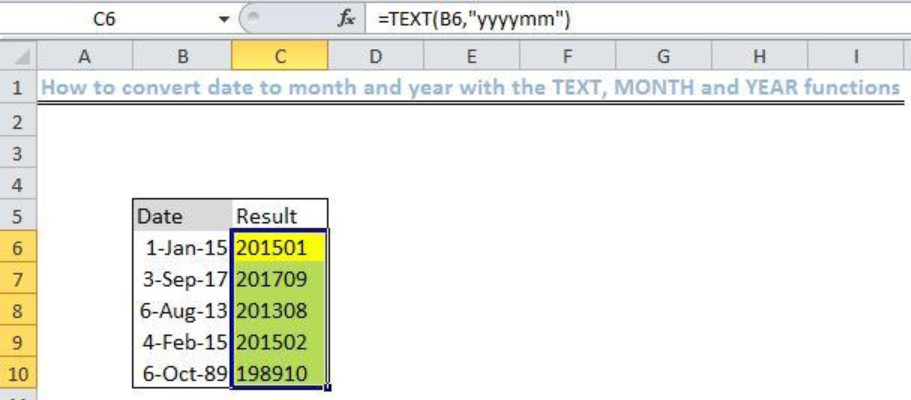Since Excel stores dates as serial numbers, this makes it simple to use them for calculations. This tutorial shows how you can add and subtract dates using basic operations in Excel. It details how to set up your data and then provides the reader with several examples.

## Get Day Name from Date

You may have a date in the distant future or the past and need to know what day of the week it falls on. This piece shows how you can accomplish this by using the TEXT, CHOOSE, or WEEKDAY functions. There are examples given for each operation.

## How to Calculate a Retirement Date

If an employee must retire at a certain age, this tutorial can show how to calculate their exact retirement date in Excel using the EDATE and YEARFRAC function. It explains how the given formula works and provides an example.

## Convert Date to Julian Format

Julian dates are often used as timestamps in programming or manufacturing. This tutorial explains how you can convert a date in Excel to the Julian date format using a combination of the RIGHT, YEAR, and TEXT functions. An example is included.

## How to Check If a Year is a Leap Year in Excel

If you need to check whether a year is a leap year in Excel, this piece shows how to accomplish this. Specifically, you use a combination of the MONTH, YEAR, and DATE functions. The formula is provided as well as an example with illustrations.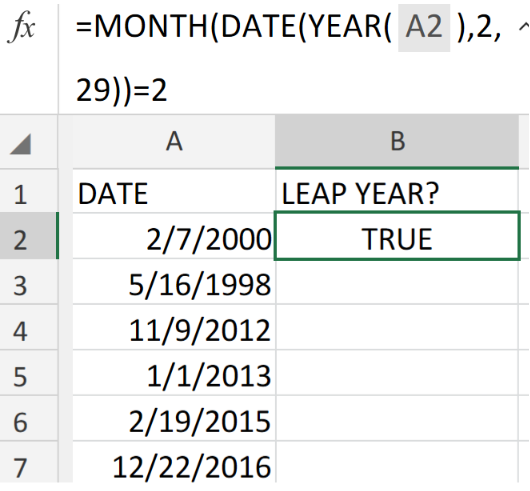## Count Holidays Between Two Dates

This piece shows the reader how to count the holidays between two given dates in Excel. You can get this done using either the COUNTIFS or the SUMPRODUCT function. There is an example and illustrations given for each operation.

## Convert Date to Text

If you have a date, such as 5/27/2019, you can convert this to the text form – May 27, 2019 – in Excel using the TEXT function. This piece shows you how to do this and provides an example with illustrations.

## Calculate Years Between Dates

Assuming you have two sets of dates, you can calculate the difference in years between those dates in a variety of ways. This tutorial shows how to accomplish this using the YEARFRAC and DATEDIF functions in Excel – with examples.

## Get Months Between Dates

Excel also allows you to calculate the number of months between two dates. This piece shows how you can do this by using the DATEDIF function. It provides the formula, an explanation for the operation, and an example with illustrations.

## Get Next Day of Week

As long as you have a start date, Excel gives you the tools you need to determine the next day of the week. This tutorial shows the reader how to do this using the WEEKDAY function. It provides the syntax of the formulas as well as an example.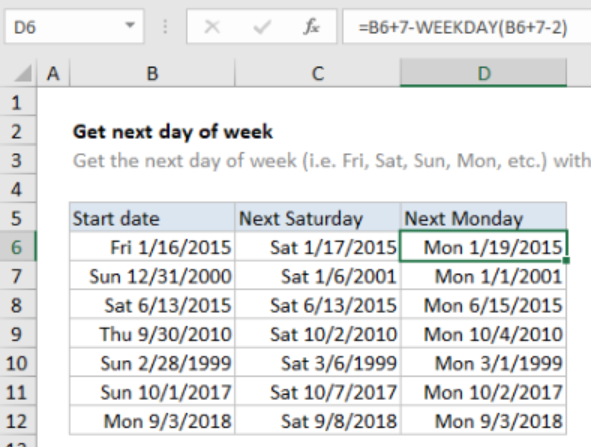If you want to add months to a particular date, you can learn how to do this here. This piece shows how to used the EDATE function to take a given date and add a set number of months to it to arrive at a new date. Examples are included.

## Get Days, Months, and Years Between Dates

This is a step-by-step guide on using the DATEDIF function in Excel to determine the length of time between two dates. It shows how this function can provide the difference in years, months, and days with the formula and examples.

## Count Day of Week Between Dates

This tutorial shows the reader how to count the days of the week between two dates by using a combination of the SUMPRODUCT, WEEKDAY, ROW, and INDIRECT functions. It includes the formula as well as an example with illustrations.

## Basic Overtime Calculation Formula

This piece explains how you can accomplish a basic overtime calculation in Excel. It doesn’t use any functions, but the formula syntax is provided along with a detailed example with illustrations to use as a guide.

## Calculate Days Remaining

This is a tutorial that guides the reader through finding the number of days remaining until a target date. In other words, it serves as a countdown formula. It uses the MAX and TODAY functions for this operation, and there is an example provided.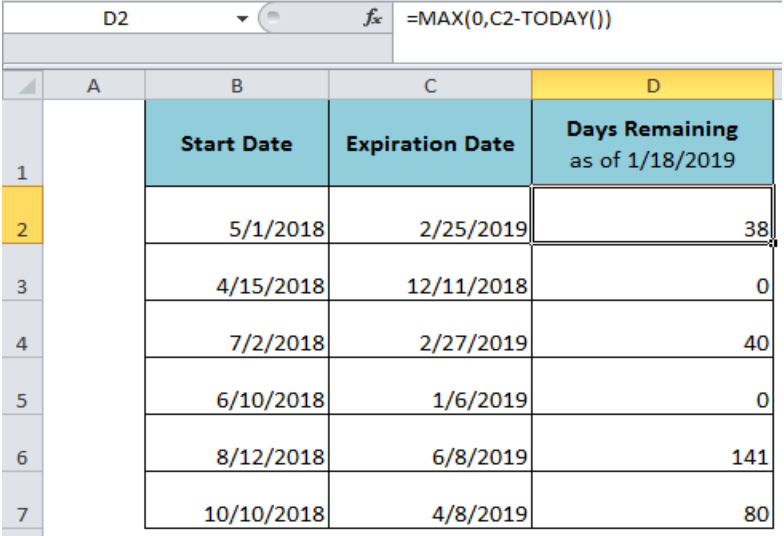## Get First Day of Month

If you need to find the first day of any given month, this piece will show you how to do this using the DAY function in Excel. It explains how the formula works and then provides the reader with an example, including illustrations.

## Get Age from Birthday

Provided you are given a birthday, Excel also gives you the means to calculate a person’s age. This tutorial shows how to accomplish this using a combination of the INT, YEARFRAC, and TODAY function. An example is included.

## How to Get Month Name from Date in Excel

If you need to isolate a month name from an entire date in Excel, this piece explains how you can do this. Specifically, you’ll use the TEXT function, and there is an example with illustrations provided as a guide.

## Net Working Day

This tutorial explains how you can find the next working day in Excel from a series of dates. It considers weekends and holidays and uses the WORKDAY function to add days to a specified date. An example is included with illustrations.

## Calculate Date Overlap in Days

If you are managing projects, you might need to find out if any dates overlap. This piece shows you how to do this by using a combination of the MAX and MIN functions in Excel. It includes an example with illustrations.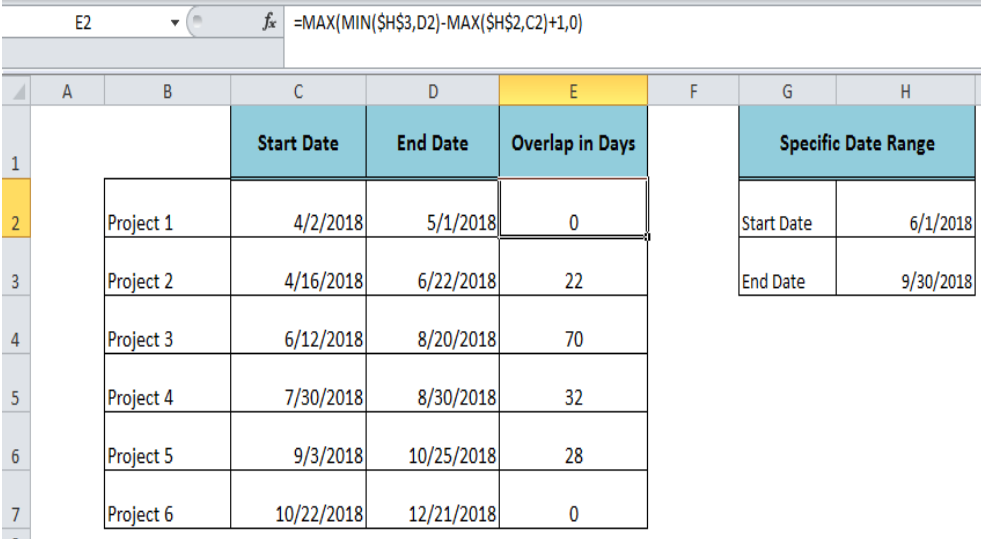## Create Date Range from Two Dates

You can create a date range from two dates in Excel using the TEXT function combined with an ampersand (&). This piece shows the reader how to accomplish this by providing the formula syntax and then giving an example with illustrations.

## Learn How to Use the DAYS Function in Excel

This is a high-level guide on using the DAYS function in Excel. It explains how you can use this function to count days between dates, provides the syntax for a formula using DAYS, and then gives several examples with illustrations.

## How to Count the Dates in the Current Month in Excel

You can quickly and automatically count the dates in a given month using a combination of the COUNTIFS, EOMONTH, and TODAY functions. This piece shows the reader how to accomplish this and includes a detailed example.

## Get Fiscal Year from Date

If your company operates on a fiscal year, this tutorial shows how you can derive the fiscal year from a list of dates. It uses a combination of the YEAR and MONTH functions with a formula. An example with illustrations is provided.

## How to Calculate the Next Anniversary Date in Excel

When you have a list of start and end dates in Excel, you can determine the next anniversary date using a combination of the EDATE and DATEDIF functions. This piece shows how to accomplish this and includes an example.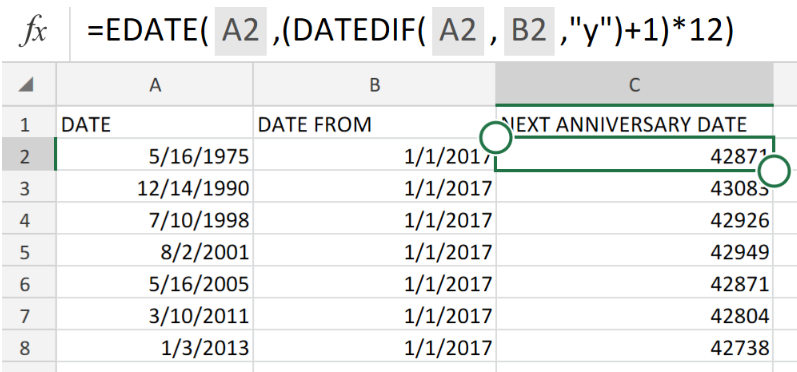## Next Biweekly Payday from Date

This tutorial illustrates how you can find the next biweekly payday from a given date. In the example, it assumes that Friday is the payday and uses the CEILING function in Excel to find the next payday. It provides the formula and illustrations as a guide.

## Custom Weekday Abbreviation

If you need to abbreviate your weekdays in Excel, this piece shows how to do this using a combination of the CHOOSE and WEEKDAY functions. It provides the general formula and then gives an example of how you can customize the abbreviations of your weekdays.

## How to Get Work Hours Between Dates in Excel

This piece is a guide for the reader on finding the work hours between two dates in Excel. In other words, you have a start date and end date, and Excel will calculate the work hours while considering weekends and holidays using the NETWORKDAYS function.

## Convert Text to Date

Excel gives you the tools to convert text to dates. The text format is generally unrecognized as a date, and this piece shows how to reformat the result using the DATE, LEFT, MID, and RIGHT functions to obtain a recognizable date in a new cell.

## Excel NETWORKDAYS.INTL Function

When you need to calculate workdays or business days excluding weekends and holidays between two dates in Excel, you can use the NETWORKDAYS or NETWORKDAYS.INTL functions. This tutorial explains the NETWORKDAYS.INTL function, which lets you customize your holidays and weekends.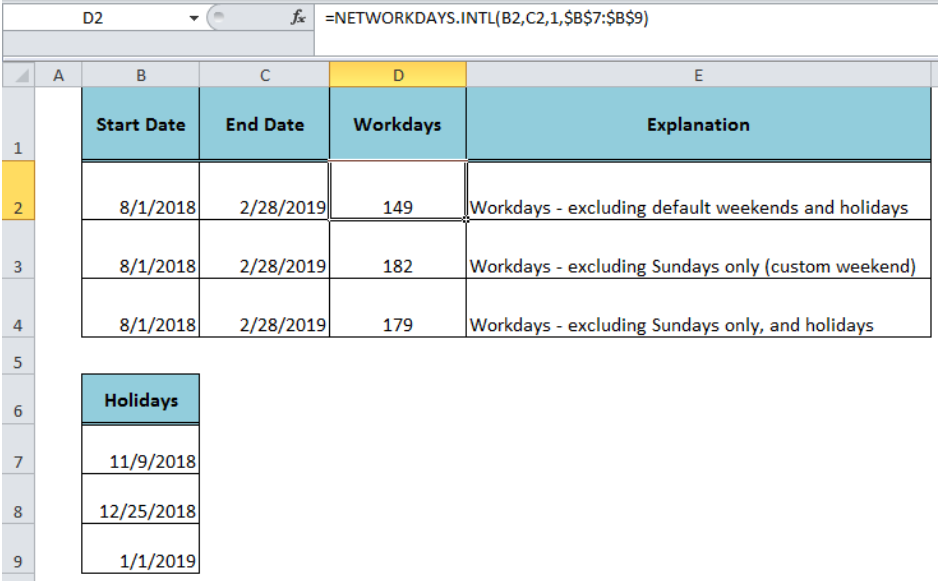## Excel EDATE Function

There are several ways to add and subtract dates in Excel, and one of the simplest is with the EDATE function. This piece explains how this is used and shows how to use this function to find due dates or maturity dates. Examples are provided.

## How to Get the nth Day of the Week in a Month in Excel

This tutorial shows the reader how to find the nth day of the week in Excel using a combination of the DAY and WEEKDAY functions. It explains how to set up your data, gives the formula syntax, and then provides an example with illustrations.

## Pad Week Numbers with Zeros

If you need to pad your week numbers in Excel (such as make week number 3 appear as “03”), this piece will show you how to accomplish this. It uses a combination of the TEXT and WEEKNUM functions, and an example is provided.

## Calculate Expiration Date

Calculating an expiration date in Excel is simple enough by simply adding your criteria to a start date. This tutorial shows the reader how to accomplish this with several different examples and scenarios. Illustrations are included as a guide.

## Get First Monday Before Any Date

This piece explains how you can use the WEEKDAY function in Excel to find the first Monday before any given date. It provides the generic formula using WEEKDAY, an explanation of how this works, and an example with illustrations.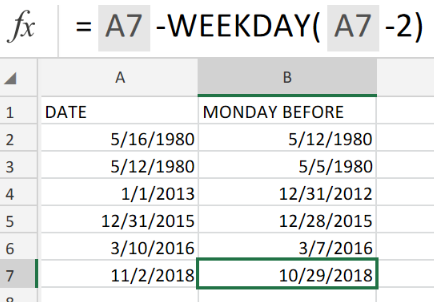## Get Quarter from Date

This tutorial shows the reader how to find the quarter from a given date in Excel. Specifically, it uses a combination of the ROUNDUP and MONTH functions to accomplish this result. Several examples are given with illustrations.

## Get Workdays Between Dates

This is another piece that details how to derive the number of workdays between two days. In the example, there is a start date and end date, and the NETWORKDAYS function is used to find the answer, which takes into account holidays and weekends.

## Date is Workday

This tutorial explains how the reader can determine whether a particular date is a workday or not. It can do this for both future and past dates, using the WORKDAY function, to determine whether listed dates are “not” holidays or weekends.

## Excel DATEVALUE Function

Excel stores dates and times as serial numbers, which allows the program to perform simple conversions and mathematical operations. The DATEVALUE function can be used to convert dates that are stored as text values into serial numbers. This piece explains how to do this.

## How to Use the Excel DAYS360 Function

In some accounting calculations, you might need to use a 360-day calendar that assumes twelve 30-day months. When doing this, you can use the DAYS360 function in Excel to return the number of days between two dates based on a 360-day calendar year.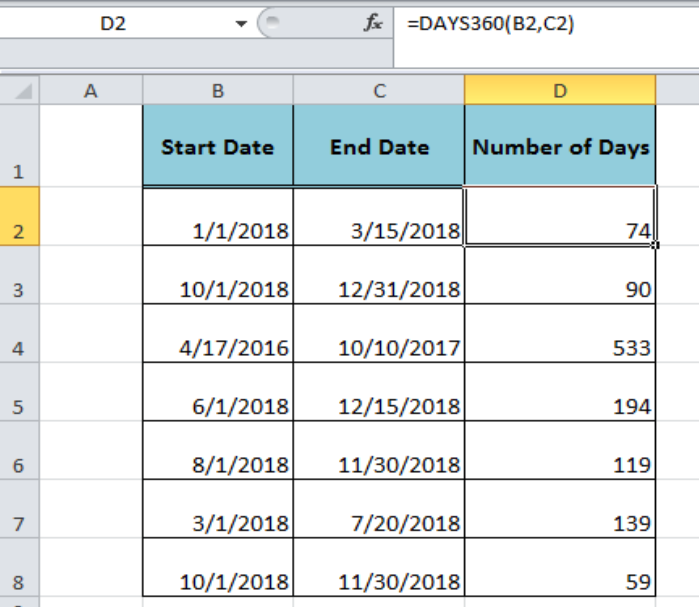## Get Last Weekday in Month

If you are scheduling a project or otherwise planning an activity, you might need to locate the last weekday in a month. This tutorial explains how you can do this using a combination of the EOMONTH and WEEKDAY functions in Excel. An example is provided.

## Count Times in a Specific Range

When working with times in Excel, you might need to find the number of cells that correspond to your criteria. This piece shows the reader how to count the number of instances of a certain time or range of times using the COUNTIFS function.

## Excel WORKDAY Function

This is a high-level piece that shows the reader how to use the WORKDAY function in Excel. It explains that this function can help you determine if a date is a “workday” or not by excluding weekends and optionally holidays. Several examples of its use are included.

## Excel EOMONTH Function

This is a tutorial on using the EOMONTH function in Excel. This piece explains that this function can help determine the last day of the month, and is also useful for finding due dates and maturity dates. It provides the syntax for the formula and several examples of its use.

## Excel YEARFRAC Function

Since there are several ways to find the number of days between two dates in Excel, this piece explains another one with the YEARFRAC function. Instead of subtracting dates, this function gives a result as the fraction of a specified year. Several examples are included as a guide.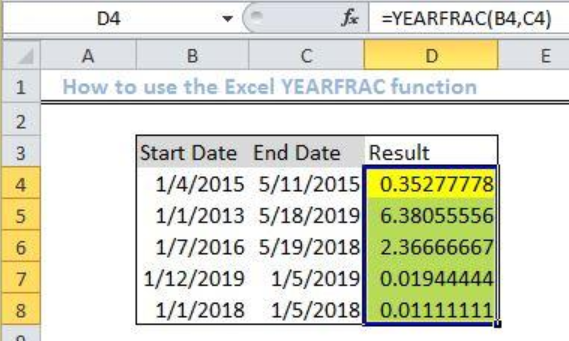## Get First Day of Previous Month

This tutorial shows how you can get the first day of the previous month using the EOMONTH function in Excel. If provides the syntax of the basic formula using EOMONTH, an explanation of the formula, and several examples with illustrations.

## How to Get the Most Recent Day of the Week in Excel

This piece explains how to find the most recent day of the week in Excel using the MOD function. Specifically, you might have a list of dates, and you can use the MOD function to find the most recent Monday’s. Several examples are provided will illustrations.

## Excel WORKDAY.INTL Function

This is a high-level piece that explains how to use the WORKDAY.INTL function in Excel. Since this function allows you to customize your weekends and holidays, this tutorial also provides the codes you can use in the formula to specify this. Examples are provided.

## Get Project Midpoint

This tutorial shows how you can derive the midpoint of a project in Excel using the WORKDAY function. It provides a formula that allows you to specify your project’s duration in days. Several examples with illustrations are included.

## Get Same Date Next Month

In your work or other activities, you might have something that needs to repeat each month. This piece shows how you can automate repeating dates with the EDATE function. It includes the syntax for the formula as well as a detailed example.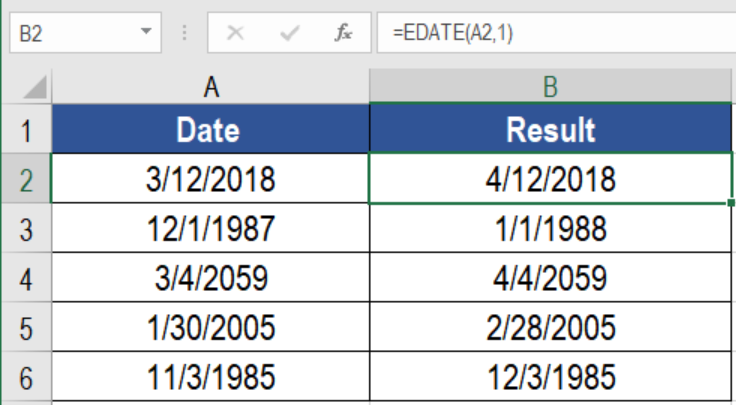This tutorial shows the reader how to add up workdays in Excel, excluding weekends and holidays. It provides several examples using both the WORKDAY and WORKDAY.INTL functions as a guide.

## Dynamic Calendar Grid

One of the things you can do in Excel is create dynamic calendars. This piece illustrates how to create a dynamic calendar grid using a combination of the CHOOSE and WEEKDAY function. It walks the reader through the process of setting up the data and includes a detailed example.

## Get Year from Date

If you have a long list of dates in Excel, you may need to extract just the years from those dates. This tutorial shows the reader how to accomplish this using the YEAR function. It includes the syntax for the formula, an explanation, and an example.

## How to Get Days in a Month Using EOMONTH and DAY Functions

This piece explains how you can get the days in a listed month using a combination of the DAY and EOMONTH functions. It includes the general and exact formulas to use as well as an example with illustrations.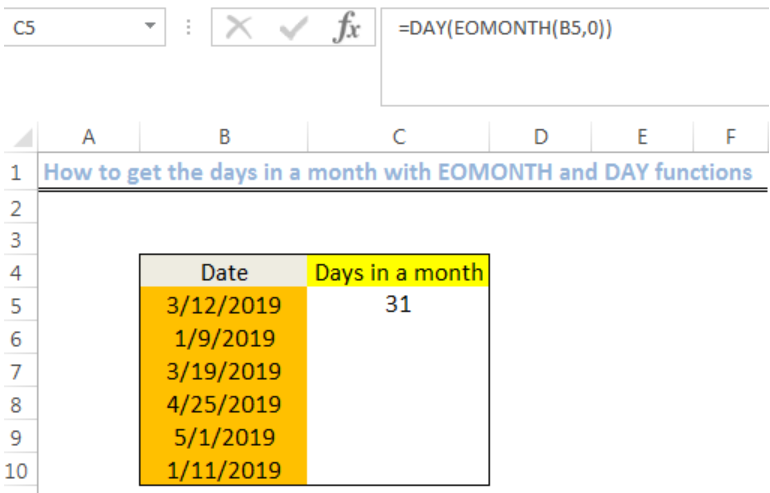## Excel YEAR Function – PENDING

This tutorial explains how to return an integer that represents the year of a given date in Excel. It uses the YEAR function to accomplish this. The formula syntax is provided, and there is also an example.

That’s a lot of Data and Time Excel tutorials. If you didn’t find what you need, or there were just too many to look through, you have other choices. Excelchat is standing by 24/7 to offer problem-specific help. Your first session is always free.